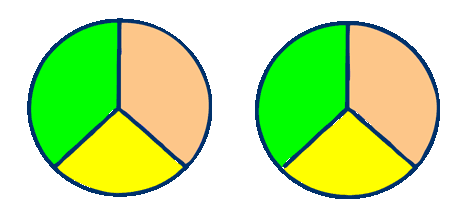# Fractions

## Proper Fractions, Improper Fractions and Mixed Numbers

So far we have been looking at fractions of one, but what happens if there is more than 1?

Lets say that we have two pizzas that we wish to share between three people.

Visualise it first - draw 2 circles and divide them both by 3.How many pieces are there? 2 green, 2 yellow and 2 orange - total 6

The total parts add up to 6 - so 6 is the denominator.

How many pieces are green, 2, - so 2 is the numerator.

The green fraction is therefore 2 out of 6, 2/6 or two sixths.

### This type of fraction, where the numerator is smaller than the denominator is known as a Proper Fraction.

_________________________________

### How could we work out fractions with a calculation?

What is 1/3 of 3? We simply divide the number by the denominator and then multiply by the numerator. 3 ÷ 3 = 1 , 1 x 1 = 1 ..... 1/3 of 3 is 1

What is 2/3 of 3, divide the number by the denominator and then multiply by the numerator. 3 ÷ 3 = 1 , 1 x 2 = 2 .... 2/3 of 3 is 2

What is 3/5 of 10, divide the number by the denominator and then multiply by the numerator. 10 ÷ 5 = 2 , 2 x 3 = 6 .... 3/5 of 10 is 6

What is 3/4 of 100, divide the number by the denominator and then multiply by the numerator. 100 ÷ 4 = 25 , 25 x 3 = 75 .... 3/4 of 100 is 75

_________________________________

What would happen if we had two pizzas and someone ate one quarter of one of the pizzas? What would we have left?Look at the diagram above, the red part has been eaten, ¼ of one pizza.

What is left (the green part)? ... 1 whole pizza and ¾ of the other. 1 and ¾ are left .... 1¾

### 2½, 4¼, 7¾ are all examples of mixed numbers

_____________________________________

What if we asked how many quarters were left? There are 4 quarters remaining in one pizza and 3 quarters remaining in the other pizza, that's 7 quarters remaining - seven more people could each have a quarter of a pizza.

We could write seven quarters as 7/4. That is still a fraction, but the numerator is greater than the denominator.

### 4/3, 12/7, 3/2 are all examples of improper fractions

_____________________________________

## Remember:

### A Mixed Number is a mixture of whole numbers and fractions.

_____________________________________